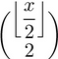# Increasing Parentheses Size in Combinations

How do you make the parentheses size of combination brackets larger?

I used the code

    \dbinom{\floor*{\dfrac{x}{2}}}{2}


and the result wasbut the parentheses weren't tall enough to cover the whole combination. Is there any way to get the parentheses to fit the height of the combination; to get the parentheses covering the floor function too? Or is this normal?

• Welcome to TeX.SX! Can you add a minimal compilable example? – egreg Feb 18 '15 at 17:54
• You get a much better result anyway with \dbinom{\floor{x/2}}{2}. As a rule, one should avoid stacked fractions in (generalized) fractions whenever possible. – egreg Feb 18 '15 at 21:42

\documentclass[oneside,12pt]{scrartcl}
$\dbinom{\floor*{\dfrac{x}{2}}}{2}$
$\left(\begin{array}{@{}c@{}} \floor*{\dfrac{x}{2}}\\2 \end{array}\right)$